# Functions and Graphs - Stage 4##### Age 14 to 16Challenge Level

In this problem we are faced with an apparently easy area problem, but it has gone horribly wrong! What happened?### Parabolic Patterns

##### Age 14 to 18Challenge Level

The illustration shows the graphs of fifteen functions. Two of them have equations y=x^2 and y=-(x-4)^2. Find the equations of all the other graphs.### Exploring Cubic Functions

##### Age 14 to 18Challenge Level

Quadratic graphs are very familiar, but what patterns can you explore with cubics?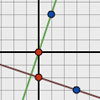### Perpendicular Lines

##### Age 14 to 16Challenge Level

Position the lines so that they are perpendicular to each other. What can you say about the equations of perpendicular lines?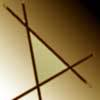### Negatively Triangular

##### Age 14 to 16Challenge Level

How many intersections do you expect from four straight lines ? Which three lines enclose a triangle with negative co-ordinates for every point ?### Curve Fitter

##### Age 14 to 18 ShortChallenge Level

This problem challenges you to find cubic equations which satisfy different conditions.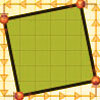### At Right Angles

##### Age 14 to 16Challenge Level

Can you decide whether two lines are perpendicular or not? Can you do this without drawing them?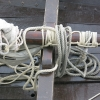### Tangled Trig Graphs

##### Age 16 to 18Challenge Level

Can you work out the equations of the trig graphs I used to make my pattern?### Back Fitter

##### Age 14 to 18Challenge Level

10 graphs of experimental data are given. Can you use a spreadsheet to find algebraic graphs which match them closely, and thus discover the formulae most likely to govern the underlying processes?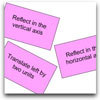### Surprising Transformations

##### Age 14 to 16Challenge Level

I took the graph y=4x+7 and performed four transformations. Can you find the order in which I could have carried out the transformations?### What's That Graph?

##### Age 14 to 18Challenge Level

Can you work out which processes are represented by the graphs?### Functions and Graphs - Short Problems

##### Age 11 to 16

A collection of short problems on straight line graphs.### Inside a Parabola

##### Age 14 to 16 ShortChallenge Level

A triangle of area 64 square units is drawn inside the parabola $y=k^2-x^2$. Find the value of $k$.### Triangular Slope

##### Age 14 to 16 ShortChallenge Level

Can you find the gradients of the lines that form a triangle?### Graph Triangles

##### Age 14 to 16 ShortChallenge Level

Use the information about the triangles on this graph to find the coordinates of the point where they touch.### Closer to Home

##### Age 14 to 16 ShortChallenge Level

Which of these lines comes closer to the origin?